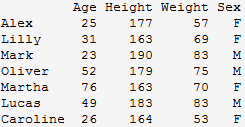# Data frame exercises

January 4, 2016
By

Want to share your content on R-bloggers? click here if you have a blog, or here if you don't.

In the exercises below we cover the basics of data frames. Before proceeding, first read section 6.3.1 of An Introduction to R, and the help pages for the `cbind`, `dim`, `str`, `order` and `cut` functions.

Answers to the exercises are available here.

Exercise 1
Create the following data frame, afterwards invert `Sex` for all individuals.Exercise 2
Create this data frame (make sure you import the variable `Working` as character and not factor).Add this data frame column-wise to the previous one.
a) How many rows and columns does the new data frame have?
b) What class of data is in each column?

Exercise 3
Check what class of data is the (built-in data set) `state.center` and convert it to data frame.

Exercise 4
Create a simple data frame from 3 vectors. Order the entire data frame by the first column.

Exercise 5
Create a data frame from a matrix of your choice, change the row names so every row says id_i (where i is the row number) and change the column names to variable_i (where i is the column number). I.e., for column 1 it will say variable_1, and for row 2 will say id_2 and so on.

Exercise 6
For this exercise, we’ll use the (built-in) dataset `VADeaths`.

a) Make sure the object is a data frame, if not change it to a data frame.
b) Create a new variable, named Total, which is the sum of each row.
c) Change the order of the columns so total is the first variable.

Exercise 7
For this exercise we’ll use the (built-in) dataset `state.x77`.

a) Make sure the object is a data frame, if not change it to a data frame.
b) Find out how many states have an income of less than 4300.
c) Find out which is the state with the highest income.

Exercise 8
With the dataset `swiss`, create a data frame of only the rows 1, 2, 3, 10, 11, 12 and 13, and only the variables `Examination`, `Education` and `Infant.Mortality`.
a) The infant mortality of `Sarine` is wrong, it should be a `NA`, change it.
b) Create a row that will be the total sum of the column, name it `Total`.
c) Create a new variable that will be the proportion of `Examination (Examination / Total)`

Exercise 9
Create a data frame with the datasets `state.abb`, `state.area`, `state.division`, `state.name`, `state.region`. The row names should be the names of the states.

a) Rename the column names so only the first 3 letters after the full stop appear (e.g. `States.abb` will be `abb`).

Exercise 10
Add the previous data frame column-wise to `state.x77`
a) Remove the variable `div`.
b) Also remove the variables `Life Exp`, `HS Grad`, `Frost`, `abb`, and `are`.
c) Add a variable to the data frame which should categorize the level of illiteracy:
[0,1) is low, [1,2) is some, [2, inf) is high.
d) Find out which state from the west, with low illiteracy, has the highest income, and what that income is.

R-bloggers.com offers daily e-mail updates about R news and tutorials about learning R and many other topics. Click here if you're looking to post or find an R/data-science job.
Want to share your content on R-bloggers? click here if you have a blog, or here if you don't.